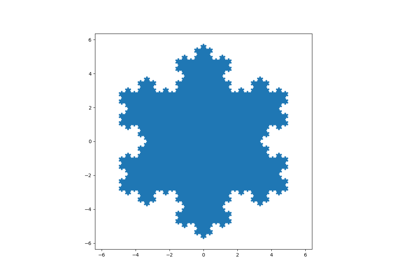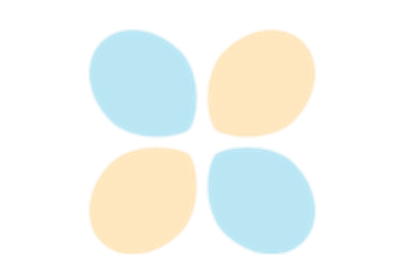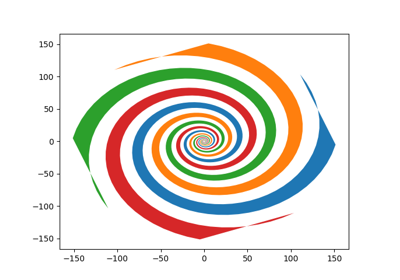# matplotlib.pyplot.fill¶

matplotlib.pyplot.fill(*args, data=None, **kwargs)[source]

Plot filled polygons.

Parameters: *args : sequence of x, y, [color] Each polygon is defined by the lists of x and y positions of its nodes, optionally followed by a color specifier. See matplotlib.colors for supported color specifiers. The standard color cycle is used for polygons without a color specifier. You can plot multiple polygons by providing multiple x, y, [color] groups. For example, each of the following is legal: ax.fill(x, y) # a polygon with default color ax.fill(x, y, "b") # a blue polygon ax.fill(x, y, x2, y2) # two polygons ax.fill(x, y, "b", x2, y2, "r") # a blue and a red polygon  data : indexable object, optional An object with labelled data. If given, provide the label names to plot in x and y, e.g.: ax.fill("time", "signal", data={"time": [0, 1, 2], "signal": [0, 1, 0]})  a list of Polygon **kwargs : Polygon properties

Notes

Use fill_between() if you would like to fill the region between two curves.

## Examples using matplotlib.pyplot.fill¶Filled polygonInteractive functionsFill Spiral